## how to modify includepath in a worksheet and an mp...

Let's say I have an mpl file and in this file there are several lines \$include<~/~/~.mpl> and in some of those called mpl files, there are also some more include lines. And assume the paths of these files are given relative to a specific folder. Now I guess this specific folder has to be mentioned by modifying includepath. But looking at the help pages related to includepath (here and here) I get this sentence "it can be modified by calling kernelopts(includepath)", but I tired typing things like kernelopts(includepath):="C:\\Homes\\testFolder"; and still I see "" for kernelopts(includepath);. How can I tell Maple to look for the files relative to this location either by typing something at the Maple worksheet before typing read("C:\\Home\\testFolder\\main.mpl); or by adding a line at my main mpl file?

## Why is it converted to a latex file and then run ...

It is said that the LaTeX export of maple2021 has been improved , but unfortunately I still encounter the following problems.

When I execute the following related graph theory code,

restart;
with(GraphTheory):
s1:=[NonIsomorphicGraphs(4,restrictto = connected,output=graphs,outputform=graph)]: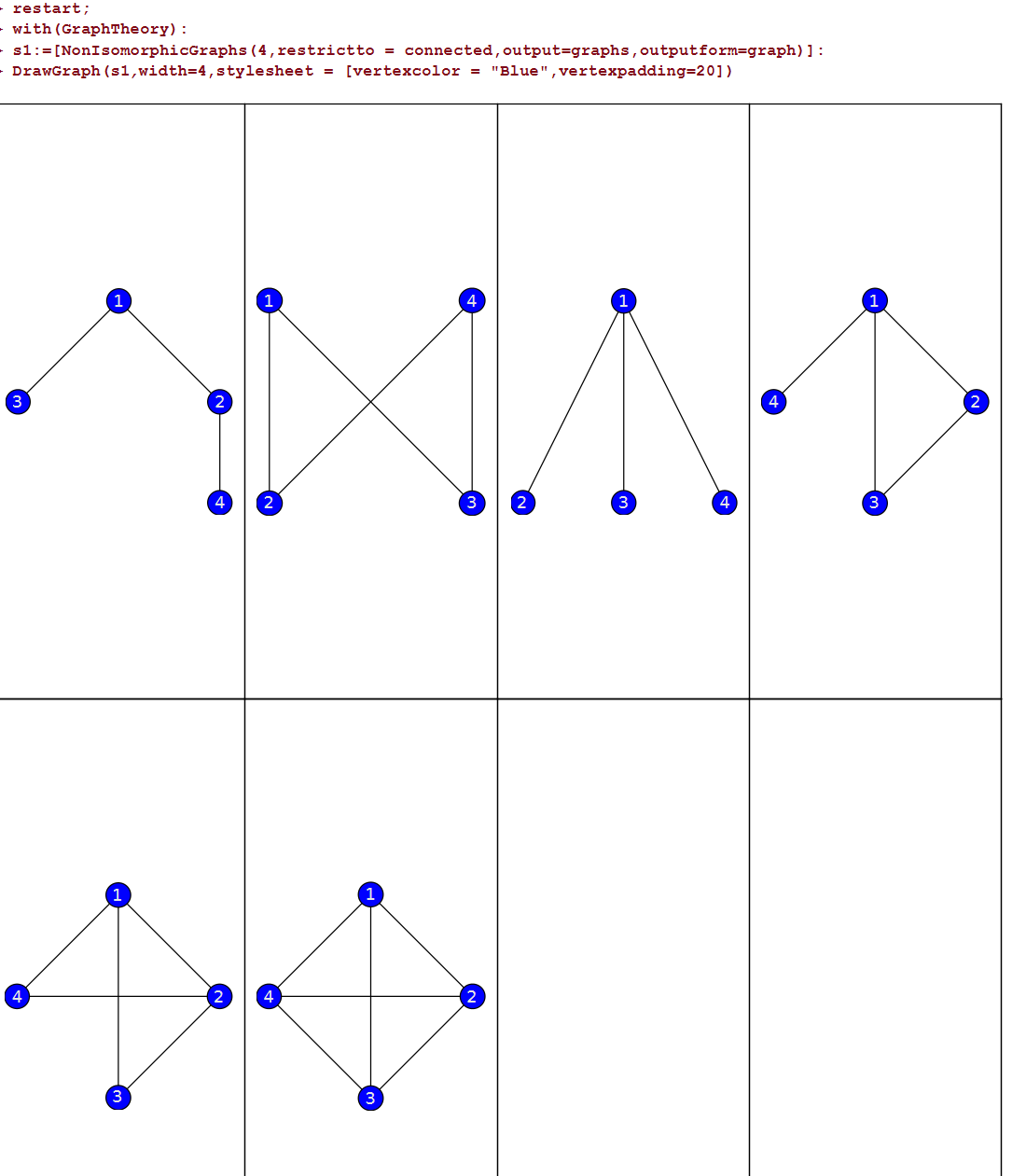It is strange that all graphs(.eps) are not produced in  corresponding folder  when I exported it as latex file . So it leads to the lack of graphs in the running results of latex.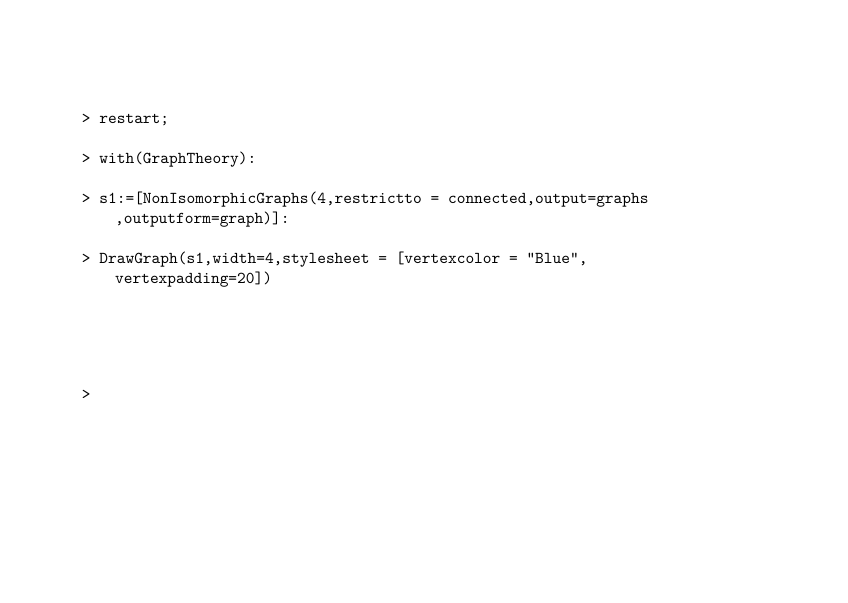If it is a single graph, there seems to be no problem.

restart;
with(GraphTheory):
s1:=[NonIsomorphicGraphs(4,restrictto = connected,output=graphs,outputform=graph)]:
DrawGraph(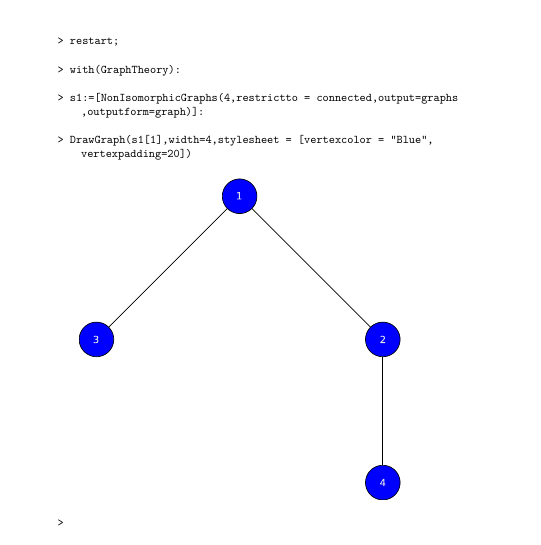graph1.mw

## Maple toolbar keeps disappearing, how do I make it...

Hi all,

When I open up Maple the toolbar which says insert: 'Math, Text etc' has disappeared. However sometimes when I restart the program the tool bar re-appears again. I have attached a screenshot have a look. I want to know is anyone else experiencing this in Maple 2021?## How do I check the equality of two equations?...

I have two equations (assignments, to be accurate) from which I know they are equal:

f__1 := sqrt(4*a^2 + lambda__g^2)*c/(2*lambda__g*a)

f__2 := c*sqrt(1/lambda__g^2 + 1/(4*a^2))

However:

testeq(f__1 = f__2) returns FAIL

verify(f__1, f__2, equal) returns  FAIL

and

simplify(f__1 - f__2, sqrt)

does not return 0 but

-c*(sqrt((4*a^2 + lambda__g^2)/(lambda__g^2*a^2))*lambda__g*a - sqrt(4*a^2 + lambda__g^2))/(2*lambda__g*a)

What is the best method for checking such equalities?

I am sorry for not using the Maple Math input option but fpr such reason it did not accept the expressions in this question. I am sure I made a mistake but I do not know what mistake.

I tested all methods mentioned above with the 1. Binominal equation sucessfully.

## Not giving the correct output...

I was trying to get an aproximation of an expression, but it doesnt seem to provide the correct answer. for the following

((12*sqrt(13))/13 - 3/4)/(1 + ((12*sqrt(13))/13)(3/4))

maple provides 0.59568 and the actual answer is 0.73744I dont know what I am doing wrong

## find a weak form to Navier equations...

I want to find a weak form to Navier equations and obtain a solution formulation . I am interested in solving this problem using a finite element solver for which we need to introduce these equations in weak form. Can anyone help me in this regard? What is a higher-order continuity in the FEM approximation. This is challenge with the FEM which is based the Lagrange basis functions. To overcome the shortcomings, should we use the isogemetric analysis being based on the NURBS basis functions? How FEM cover this shortage for solving this type of equations?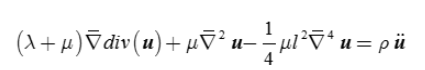## Is it possible to write a table type together with...

We can write a list, set, MutableSet and array type together with their entries types. For example list(polynom) or array(array(integer)). But what about a table? For example how can I emphasize a type table with indices of the type integer and entries a list of integers? I was guessing table(integer,list(integer)) which is not working, so my guess is not correct. I tried some other combinations which they didn't work too. I can't see anything in the programming guide and the help of Maple or a post here that is addressing this question.

Example:

test:=table([1=[1,2,3],2=[6,5,4]]):
type(test,table(integer,list(integer))): # which of course is not working.

## How to remove several entries from an array effici...

Consider 1-dimensional arrays in Maple. To add one element at the end of an array, there is a pre-defined command ArrayTools:-Append (or using ArrayTools:-Insert if one wants to add an element at another location of the array) and to remove one entry from an array there is another pre-defined command ArrayTools:-Remove. Now let's say I want to add several elements and remove several elements. If I want to add all of the new elements at the end of the array, there is a predefined command ArrayTools:-Extend. If I want to remove several entries that are sequal, then I can give the second argument of ArrayTools:-Remove as a range n1..n2. But now let's say I want to remove several entries that are not sequal, for examples entries with indices in [5,8,14]. Is there any special efficient way to do it or just defining a loop to do the removals one by one? Removing by a loop is not very nice since the number of indices will change after each removal. Then another idea is to redefine the whole array using a select or a seq. But I'm guessing that none of these are the best way to do so. If I was dealing with MutableSet, I would use &minus, but I can't use MutableSets in every situation, because MutableSets change the order of elements and have more special properties like no repeated elements etc.

## Lack of parentheses in displaying substitution in ...

Hi there.

I noticed some little bug:

example.mw

As you can see the second term in serie C looks like -M/sqrt(-M^2 + E) - k, but not like M*k/sqrt(-M^2 + E).

## simplify/symbolic...

Hi there.

It looks strange but simplify/symbolic cannot handle expression in file:

example.mw

Only after expanding numerator and denominator by hand.

I think simplify/symbolic should be smarter.

## Problems integrating Bessel functions...

Hi folks

I'm trying to integrate products of Bessel functions and reproduce known results in the literature. For example, the integralshould evaluate to 1/q if q>k and 0 if q<k, but maple only gives the first solution, even when 'assuming q<k' is specified. It should give 1/2q when k=q -- which it does but only when I put k=q in the integral. For some reason, it always assumes q>k whatever I do.

There's a lot of examples like this. Am I missing something? Is there a way to return an answer with all the (correct!) solutions?

## Maple latex uses command that gives error...

Using latest TeXLive distribution, and current Maple 2021 maple.sty file, Maple generated Latex uses command called

\pdfstringdefDisableCommands

which is unknown. I found this when I was trying to compile latex generated from Student:-Calculus1:-ShowSolution command.

The above latex command is called/used in maple.sty file, but it is not defined anywhere outside. It might have been defined many years ago?  I only see one reference to it in an old posting at tex stackexchange from few years ago.

This is how to reproduce this error (one needs to have latex installed on your PC to compile the file). On windows MikTeX can be used. On Linux, TeXLive.

restart;
the_output:=Student:-Calculus1:-ShowSolution(Int(x^3,x=0..1));
latex(the_output)


Then copied the latex, and pasted it in my latex file

\documentclass{article}
\usepackage{amsmath}
\usepackage{array}
\usepackage{maple}% set the path to reach this in Maple etc/ folder in its installation
\begin{document}

$\begin{array}{ccc} & {} & \textrm{Integration Steps} \\ {} & {} & \int_{0}^{1}x^{3}d x \\ \textrm{▫} & {} & \textrm{1. Apply the}\textrm{power}\textrm{rule to the term}\int x^{3}d x \\ {} & \textrm{◦} & \textrm{Recall the definition of the}\textrm{power}\textrm{rule, for n}\textrm{≠}\textrm{-1} \\ {} & {} & \int x^{n}d x =\frac{x^{n +1}}{n +1} \\ {} & \textrm{◦} & \textrm{This means:} \\ {} & {} & \int x^{3}d x =\frac{x^{3+1}}{3+1} \\ {} & \textrm{◦} & \textrm{So,} \\ {} & {} & \int x^{3}d x =\frac{x^{4}}{4} \\ {} & \textrm{◦} & \textrm{Apply limits of definite integral} \\ {} & {} & \frac{x^{4}}{4}{\raisebox{-0.36em}{\Big |}}{\mstack{}{_{x \hiderel{=}1}}}-\left(\frac{x^{4}}{4}{\raisebox{-0.36em}{\Big |}}{\mstack{}{_{x \hiderel{=}0}}}\right) \\ & {} & \textrm{We can rewrite the integral as:} \\ {} & {} & \frac{1}{4} \end{array}$

\end{document}

And now when compiling the above using the latex compiler, it gives this error

(base) >pdflatex foo5.tex
This is pdfTeX, Version 3.14159265-2.6-1.40.21 (TeX Live 2020) (preloaded format=pdflatex)
restricted \write18 enabled.
entering extended mode
(./foo5.tex
LaTeX2e <2020-10-01> patch level 2
L3 programming layer <2020-12-07> xparse <2020-03-03> (/usr/local/texlive/2020/texmf-dist/tex/latex/base/article.cls
Document Class: article 2020/04/10 v1.4m Standard LaTeX document class
(/usr/local/texlive/2020/texmf-dist/tex/latex/base/size10.clo)) (/usr/local/texlive/2020/texmf-dist/tex/latex/amsmath/amsmath.sty
For additional information on amsmath, use the ?' option.
(/usr/local/texlive/2020/texmf-dist/tex/latex/amsmath/amstext.sty (/usr/local/texlive/2020/texmf-dist/tex/latex/amsmath/amsgen.sty)) (/usr/local/texlive/2020/texmf-dist/tex/latex/amsmath/amsbsy.sty) (/usr/local/texlive/2020/texmf-dist/tex/latex/amsmath/amsopn.sty)) (/usr/local/texlive/2020/texmf-dist/tex/latex/tools/array.sty) (/mnt/g/public_html/styles/maple.sty
Package: maple 2005/03/17 v1.16
Defining Maple Utility Macros
Defining Maple Plot Environemnts
...Defaults to "dvips" Driver
(/usr/local/texlive/2020/texmf-dist/tex/latex/graphics/epsfig.sty (/usr/local/texlive/2020/texmf-dist/tex/latex/graphics/graphicx.sty (/usr/local/texlive/2020/texmf-dist/tex/latex/graphics/keyval.sty) (/usr/local/texlive/2020/texmf-dist/tex/latex/graphics/graphics.sty (/usr/local/texlive/2020/texmf-dist/tex/latex/graphics/trig.sty) (/usr/local/texlive/2020/texmf-dist/tex/latex/graphics-cfg/graphics.cfg) (/usr/local/texlive/2020/texmf-dist/tex/latex/graphics-def/dvips.def))))
Defining Automatic Style Generation Macros
! Undefined control sequence.
l.3248 \pdfstringdefDisableCommands
{\let\(\fakemath}    % 2.
?`

The above command is on line 3248 in maple.sty file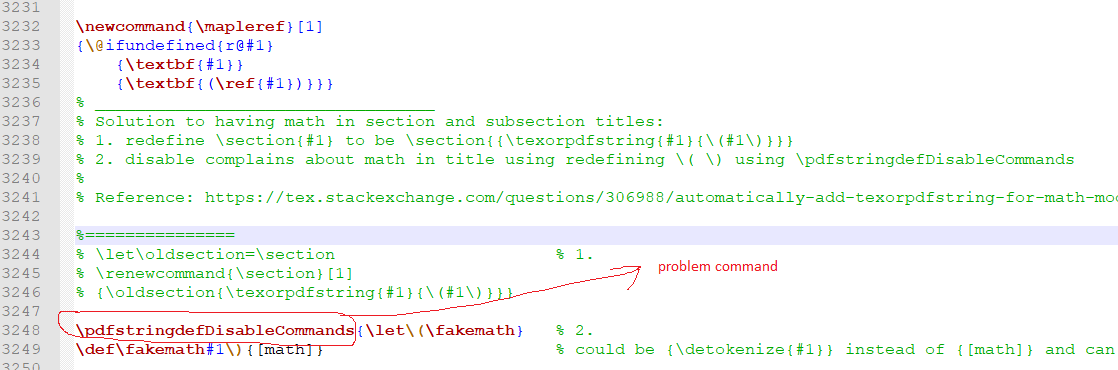I tried to workaround it, but do far could not find a way.

Is there a way to fix this problem in Maple 2021 latex?

## Any automatic style editing like pylint for maple ...

Is there any predefined command in Maple that you can run it on a Maple code file such as an mpl file, or just on a piece of code written in the worksheet file and it edits the code by the easy usual styling preferences such as what pylint does in Python? Adding spaces arround ":=" or adding indentation etc.

Hi.

I experience "broken links" in Maple. That is when I've used the the search field Alt+S or visited the help pages, very often thereafter I have to restart Maple in order to use e.g. the Help again, a task or whatever is essentially a link. Is this a known problem in Maple and if what do I do remedy it.